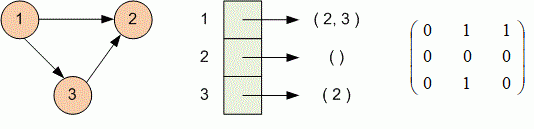Problems

Time limit 1 second
Memory limit 128 MiB

Simple directed graph is given by adjacency list. Print its representation in the form of adjacency matrix.

## Input data

The first line contains the number of vertices n (1n100). Then n lines are given. The i-th line contains the description of all edges, outgoing from the i-th vertex. Description starts with the number of outgoing edges. Then given the vertex numbers where the edges go. All vertices are numbered from 1 to n.

## Output data

Print the adjacency matrix of oriented graph.## Examples

Input example #1
3
2 2 3
0
1 2

Output example #1
0 1 1
0 0 0
0 1 0